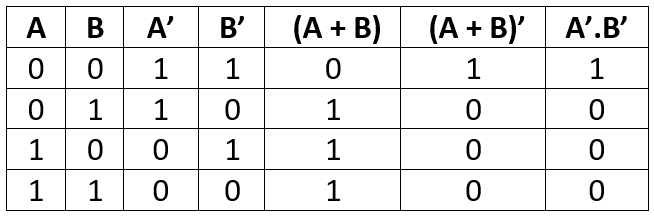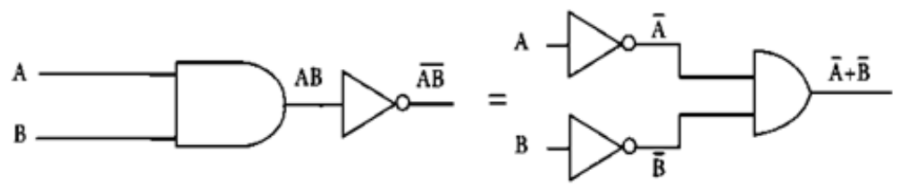De Morgan's Law# First Theorem
﻿It states that “The complement of a sum equals to the product of the complements.”
i.e.,
➡ (A + B)’ = A’.B’﻿# Second Theorem

It states that “The complement of a product is equal to the sum of the complements.”
i.e.,
(A.B)’ = A’ + B’